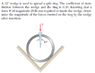# Vector Mechanics Statics question

ashishsinghal
In the question I am not able to understand what the scenario is. Is it that we are removing the P force after insertion? If that is the case, both the normal and the friction forces will change after removal of P. Then how can we calculate.

#### Attachments

•Capture.PNG
10.5 KB · Views: 522

Homework Helper
It reads that the downwards P force has been removed - then there would be a net restoring force upwards on the wedge equal to P which is balanced by the static friction.

You need to work out what the horizontal components the force has to be to make the friction force big enough to do that. Solving it requires understanding of how static friction works.

Last edited by a moderator:
Homework Helper
Gold Member
2022 Award
There's more to it than that. First need to work out what the normal force is during the last little part of the insertion. Bear in mind that that force is normal to the cut surface, not normal to P. So be careful in calculating the (peak) force that P has to match.
Having determined that normal force, you can calculate the maximum frictional force that is available to prevent the wedge slipping back out, and how much force is needed to achieve that. Note that you should not assume the wedge stays put.

Last edited by a moderator:
ashishsinghal
There's more to it than that. First need to work out what the normal force is during the last little part of the insertion. Bear in mind that that force is normal to the cut surface, not normal to P. So be careful in calculating the (peak) force that P has to match.
Having determined that normal force, you can calculate the maximum frictional force that is available to prevent the wedge slipping back out, and how much force is needed to achieve that. Note that you should not assume the wedge stays put.

But wouldn't the normal force change once P has been removed?

Studiot
Note that you should not assume the wedge stays put.

Why not? : You have no information that it moves or about the coefficient of sliding friction.

You should assume that 25 lbs is just sufficient to place the wedge where you see it and that it remains there in static equilibrium.

Otherwise the problem cannot be solved with the information provided.

Homework Helper
Gold Member
2022 Award
But wouldn't the normal force change once P has been removed?
I think you do have to assume that the split ring is fairly elastic, so the 'closing' force it exerts is the same, for a given degree of opening, whether being forced open or being allowed to close a little.
Studiot said:
You have no information that it moves or about the coefficient of sliding friction.
No, but it's the static friction that matters to prevent the ring starting to close again, and we do know that.

Studiot
No, but it's the static friction that matters to prevent the ring starting to close again, and we do know that.

Ergo it doesn't move.